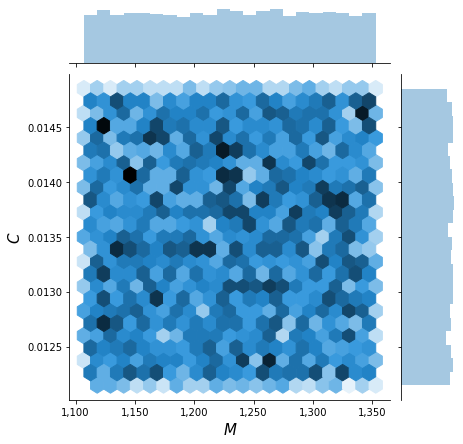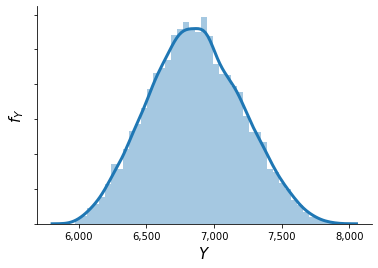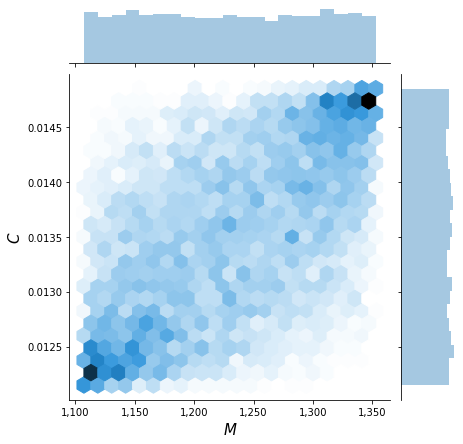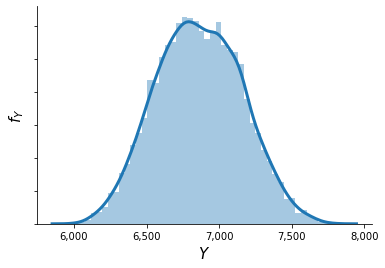# Uncertainty propagation¶

We show how to conduct uncertainty propagation for the EOQ model. We can simply import the core function from temfpy.

:

import matplotlib.pyplot as plt
import matplotlib as mpl
import seaborn as sns
import chaospy as cp

from temfpy.uncertainty_quantification import eoq_model
from econsa.correlation import gc_correlation


## Setup¶

We specify a uniform distribution centered around $$\mathbf{x^0}=(M, C, S) = (1230, 0.0135, 2.15)$$ and spread the support 10% above and below the center.

:

marginals = list()
for center in [1230, 0.0135, 2.15]:
lower, upper = 0.9 * center, 1.1 * center
marginals.append(cp.Uniform(lower, upper))


## Independent parameters¶

We now construct a joint distribution for the the independent input parameters and draw a sample of $$1,000$$ random samples.

:

distribution = cp.J(*marginals)
sample = distribution.sample(10000, rule="random")


The briefly inspect the joint distribution of $$M$$ and $$C$$.

:

plot_joint(sample)We are now ready to compute the optimal economic order quantity for each draw.

:

y = eoq_model(sample)


This results in the following distribution $$f_{Y}$$.

:

plot_quantity(y)## Depdendent paramters¶

We now consider dependent parameters with the following correlation matrix.

:

corr = [[1.0, 0.6, 0.2], [0.6, 1.0, 0.0], [0.2, 0.0, 1.0]]


We approximate their joint distribution using a Gaussian copula. This requires us to map the correlation matrix of the parameters to the correlation matrix of the copula.

:

corr_copula = gc_correlation(marginals, corr)
copula = cp.Nataf(distribution, corr)


We are ready to sample from the distribution.

:

sample = copula.sample(10000, rule="random")


Again, we briefly inspect the joint distribution which now clearly shows a dependence pattern.

:

plot_joint(sample):

y = eoq_model(sample)


This now results in a distribution of $$f_{Y}$$ where the peak is flattened out.

:

plot_quantity(y)